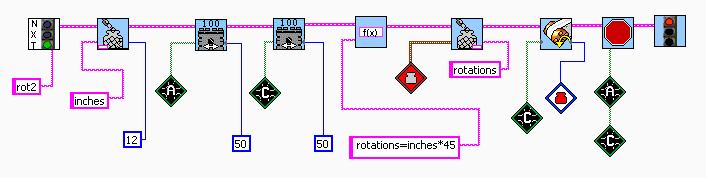Question 4: Change your program so that you can set the distance in inches instead of degrees of rotation. You will need to define a variable and set it to the number of inches you want the robot to go. You need a function that describes how many rotations the motor needs to turn to go 1 inch. The result of the calculation is called rotations and is put in the red container. The value in the red container is used to set the number of rotations for the motor.

 To solve problem 4, you have to define a variable called inches and set it to the number of inches you want the robot to go. You need a function that describes how many rotations the motor needs to turn to go 1 inch. The result of the calculation is called rotations and is put in the red container. The value in the red container is used to set the number of rotations for the motor.Question 5 All Questions